# Reflection Attenuators

New content for July 2009! This page now provides more details on designing reflection attenuators.

A reflection attenuator uses a four-port quadrature coupler, with two matched terminations on the coupled and through ports. The terminations have to present at least a partially real impedance for the device to work.

Reflection attenuators can be continuously variable, or "digital", with two or more discrete states. Most often they are used as variable phase shifters. Depending on the type of coupler uses they can provide an octave bandwidth, and with careful design they can provide phase invariant response. By phase invariant, we mean that the transmission angle (angle S21) does not change very much with attenuation. A good figure of merit might be an attenuator that provides 10 dB range, with only 10 degrees of phase run-out.

There are two solutions for the matched terminations for a reflection attenuator, one for RT>Z0, and one for RT<Z0:

RT>Z0: RT=Z0*(10^(dB/20)+1)/(10^(dB/20)-1)

RT<Z0: RT=Z0*(10^(dB/20)-1)/(10^(dB/20)+1)

Put away your calculator, we have an on-line attenuator calculator that will do the heavy lifting for you. Note that in our equations dB values are positive, 1 dB means that transmission coefficient =-1 dB. If you normalized to Z0=1 ohm, the two solutions are reciprocal.

Below are the computed values for RT for decibel values 1 t0 20 dB for lossless coupler, with Z0=50 ohms, and values rounded to three significant digits. You don't need four digits of accuracy, nothing in microwave engineering requires such precision.

dB RT>Z0 (ohms) RT<Z0 (ohms)
1 870 2.88
2 436 5.73
3 292 8.55
4 221 11.3
5 178 14.0
6 150 16.6
7 131 19.1
8 116 21.5
9 105 23.8
10 96.2 26.0
11 89.2 28.0
12 83.6 29.9
13 78.8 31.7
14 74.9 33.4
15 71.6 34.9
16 68.8 36.3
17 66.4 37.6
18 64.4 38.8
19 62.6 39.9
20 61.1 40.9

### Reflection attenuator using coupled lines

The coupled line coupler is an excellent choice for a quadrature coupler. In real life, the best implementation of this would be in stripline, but a pretty good coupler can be made on microstrip using the Lange topology. The beauty of a coupled-line is that the phases between the two split ports will be 90 degrees automatically.

The ideal values for even and odd mode for 3 dB coupling are Ze=121.5, and Zo=20.6 ohms, which we entered in the model.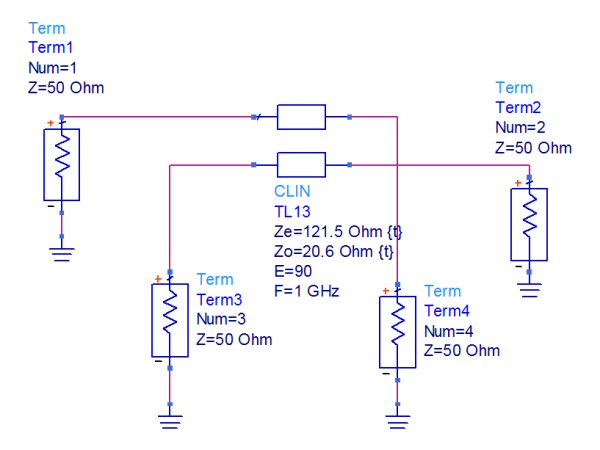Below are the transmission coefficients of the two "output" ports, 3 and 4. Note the ~3 dB value at center frequency.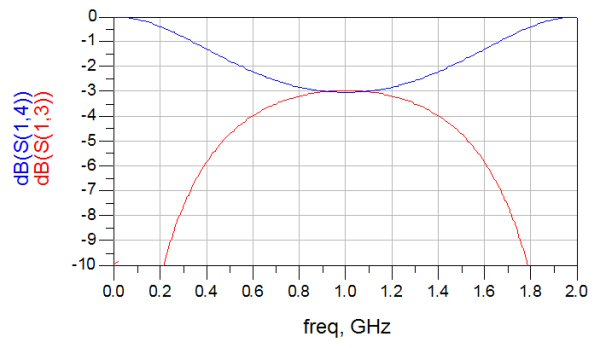We didn't even plot the phase angle between the two output ports, because it is always 90 degrees. Here's the isolation response, and one of the reflection coefficients. If we didn't round off the impedances slightly these would be perfectly matched.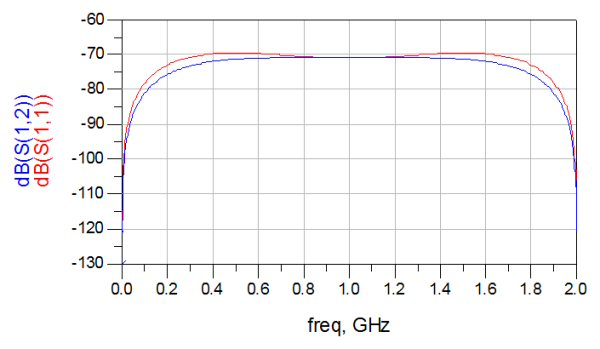Now we will use the coupler to create two reflection attenuators. The upper one uses the solution for RT>Z0 (the R1 equation), while the lower one uses the solution for RT<Z0 (the R2 equation). We've put the equations into the analysis so that dB values can be entered instead of RT values. Note that the way the coupler is used, a crossover is needed, which is not usually a problem, but in practice can contribute to phase errors.

By the way, there is no need to plot the predicted phase of a coupled line coupler, it is 90 degrees at all frequencies!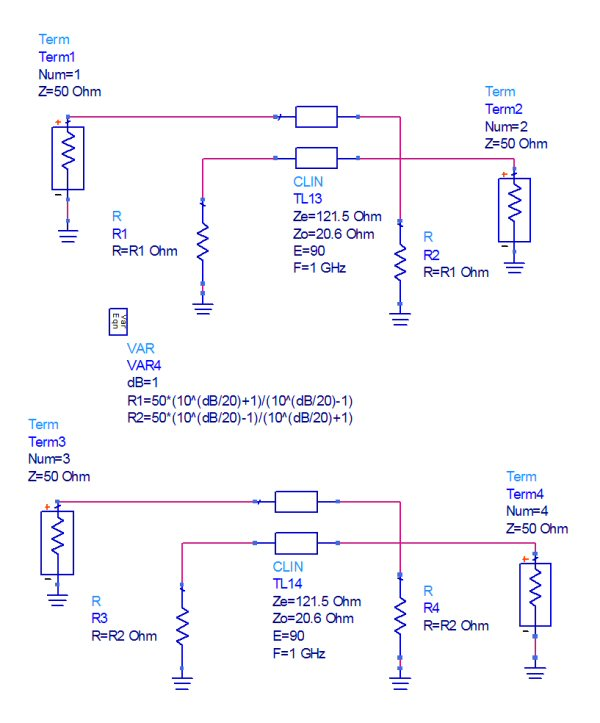Here's the transmission coefficients of both attenuators, with the attenuation value swept from 1 to 20 dB. If we had perfectly solved for the even and odd-mode impedances for even power split, they would be identical and you would only see one line color.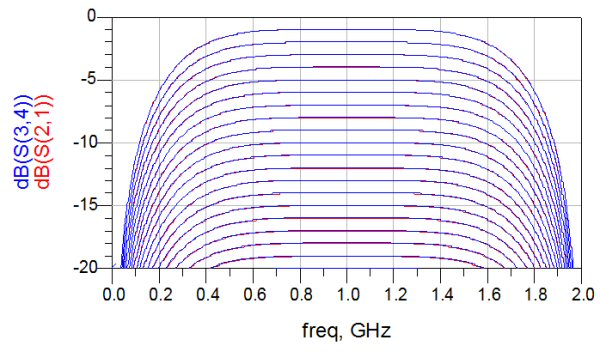Here's the reflection coefficients of both attenuators, with the attenuation value swept from 1 to 20 dB. If we had perfectly solved for the even and odd-mode impedances for even power split, they would be identical. Therefore there is no obvious advantage for one of the two types.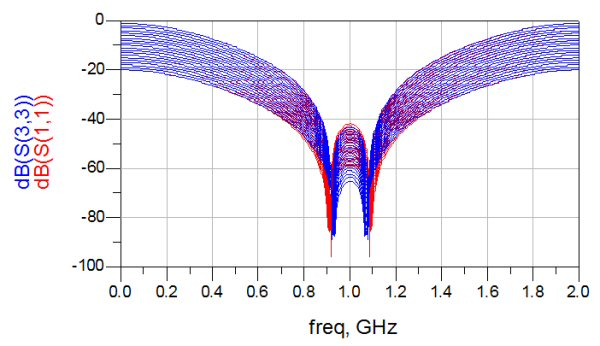Below we have narrowed up the bandwidth of the analysis to just 900 MHz to 1100 MHz. This illustrates the phase of S21 as you kick in attenuation (it's a polar plot, not a Smith chart). The phase is maintained at any attenuation value, except it is flipped 180 degrees as you pass through the center of the Smith chart. This property of the reflection attenuator is useful if you want to create a bi-phase modulator, or a vector modulator. We'll be discussing these networks soon.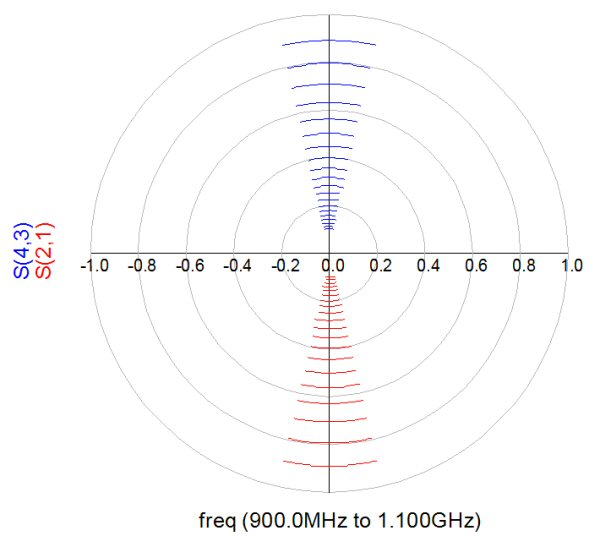### Refection attenuator using branchline coupler

We'll repeat the previous analysis using a branchline coupler to provide quadrature split. And you will see a great example of why the branchline is to be avoided, it has very limited bandwidth! Below is an ideal branchline coupler in fifty ohms. Ports 3 and 4 are the coupled and thru ports, while port 2 is the isolated port. Port 3 is not "coupled" in the sense of a coupled line, but it is the port that serves the same purpose.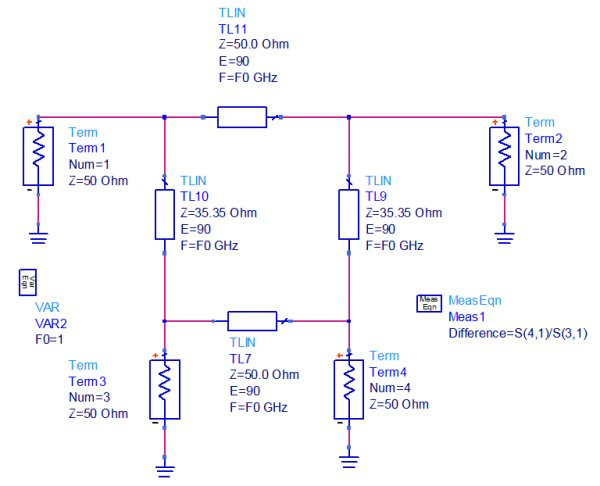The responses of the coupled and thru ports is quite narrow.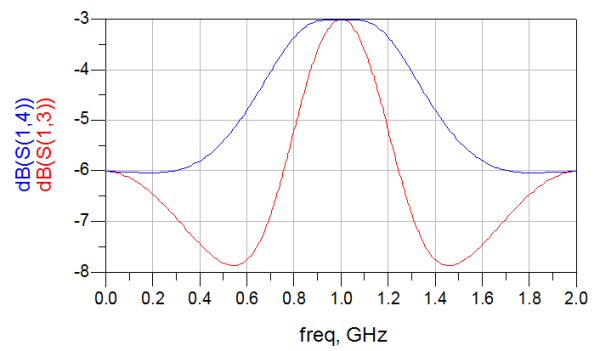And so is the match and isolation.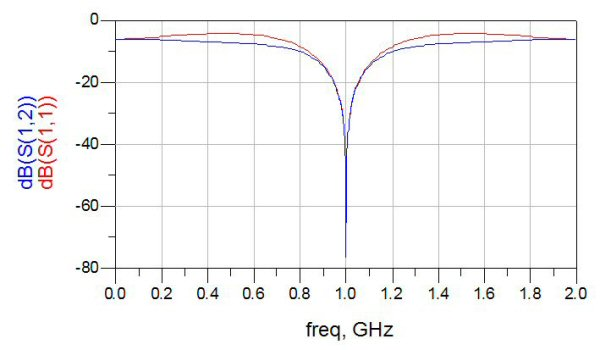Here's the phase difference between the coupled and thru ports, it provides the ideal 90 degree response at the center frequency but quickly falls apart as you move in either direction.Now it's time to create a variable attenuator. Below we have created two, one where RT>Z0, and one where RT<Z0.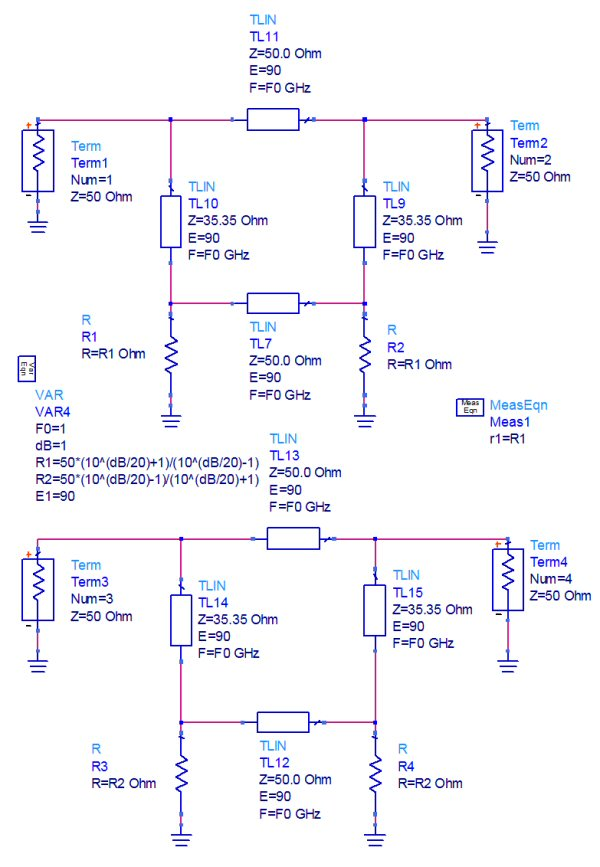Here the two responses as we step them from 1 to 20 dB. Quite a pretty graph, but only because of the huge variation across frequency. It is obvious that the RT<Z0 is the better choice. Maybe you could use this structure over 5 or 10% bandwidth. The RT>Z0 should only be used at a single frequency.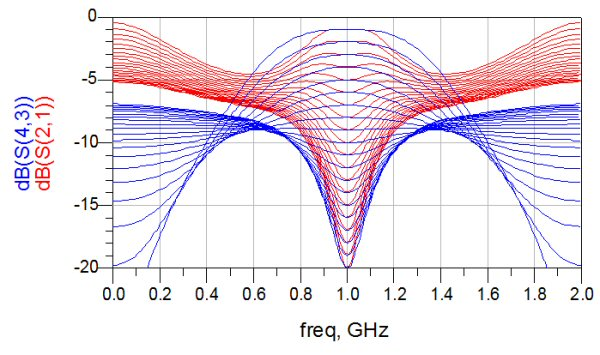Here's the impedance match at the same attenuation steps.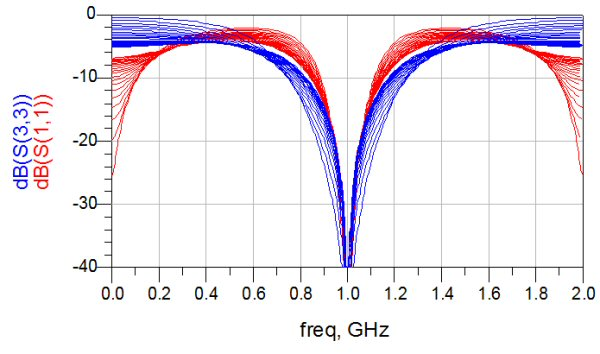Now let's look at the transmission angle trajectory. At 900 MHz, the phase has dramatic error from the ideal value (look for the four vector arrows).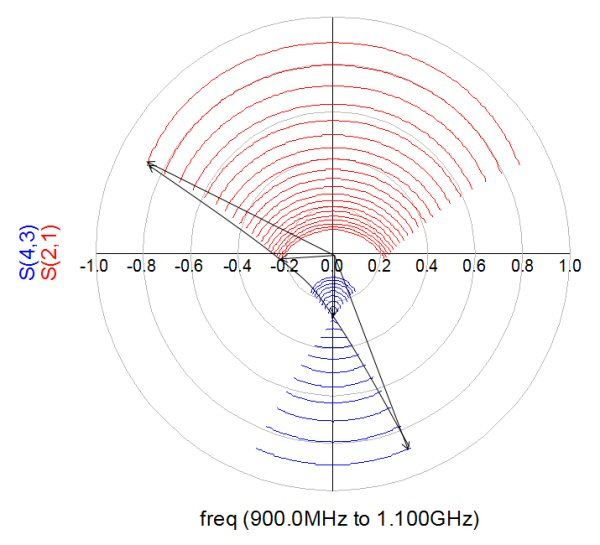In summary, don't use the branchline version of the reflection attenuator if you can help it! If you are stuck on microstrip, consider using a Lange.

### Terminations for reflection attenuators

You can create variable resistances for reflection attenuators using PIN diodes, or FETs. We'll cover this topic soon.

Author : Unknown Editor